## 8.4Flow around a cylinder

Flow around a cylinder was used as an example of boundary layer separation in Sec. 6.5 . It showed an image of vortices shedding from the cylinder in a periodic manner, known as the Kármán vortex street.

The image comes from a CFD simulation in two dimensions, representative of a cylinder of inﬁnite axial length, using the principal parameters: cylinder diameter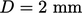; freestream velocity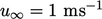in the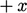-direction; and, ﬂuid kinematic viscosity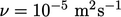. The corresponding Reynolds number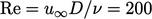, which falls within the laminar ﬂow regime.

The computational mesh used in the simulation is described in Sec. 8.3 . The centre height of the cells adjacent to the cylinder was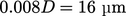, corresponding to a calculated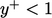.

The simulation used the transient solution algorithm in Sec. 5.19 , solving for momentum conservation for an incompressible ﬂuid, with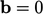and constant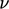. No energy equation was solved and no turbulence modelling was required since the ﬂow was laminar.

The freestream boundary conditions from Sec. 4.16 were applied to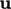and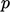over the entire external boundary, with reference values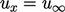,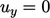and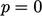. The no-slip condition,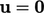and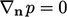, was applied on the cylinder boundary.

The simulation ran forwith a time step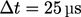using recommended numerical schemes from Sec. 3.23 . Oscillations began in the wake of the cylinder at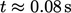, soon leading to shedding of vortices which reached a stable pattern at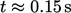.

The following ﬁgure, shaded by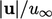, shows the slower-moving vortices as the darker structures which propagate downstream of the cylinder.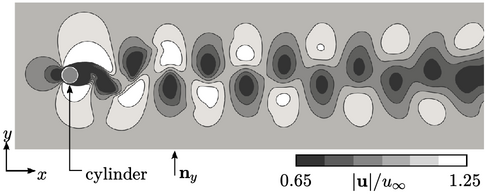The repeated vortex shedding causes oscillations in the force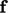of the ﬂuid on the cylinder. The force is calculated as the sum of viscous and pressure forces acting on the cylinder patch faces (), in kinematic units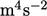(from kinematic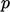and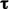) by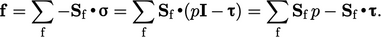(8.1)
The dimensionless lift coeﬃcient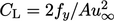is shown below, calculated from: the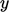-component of the force is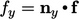, where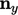is the unit vector in the-direction; and, the projected frontal area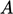of the cylinder.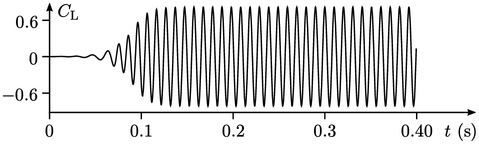The oscillation period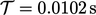corresponds to a Strouhal number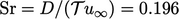, consistent with published data.1

1Christoﬀer Norberg, Flow around a circular cylinder: Aspects of ﬂuctuating lift, 2001.

Notes on CFD: General Principles - 8.4 Flow around a cylinder